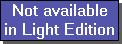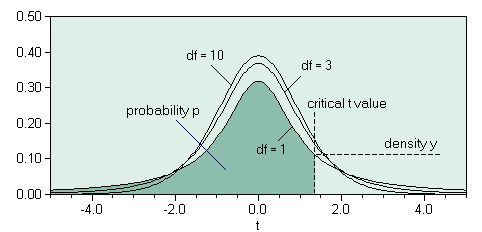The SDL Component Suite is an industry leading collection of components supporting scientific and engineering computing. Please visit the SDL Web site for more information....## tDistriQuantileUnit: SDL_statis Class: none Declaration: function tDistriQuantile (p: double; df: integer): double;

The function tDistriQuantile returns the value of the quantile of the t-distribution for a given probability p, and a specified number of degrees of freedom ix. The parameter p may take any value in the open interval (0,1). The parameter ix may take values between 1 and MaxInt. If tDistriQuantile is called using invalid arguments a value of 0.0 is returned.Hint 1: The calculated value is exact to about 8 significant figures.

 Hint 2: The function tDistriQuantile calculates the quantile by using the integral from 0.0 to the t value. This differs from the convention of how the integral of the t distribution is used in many statistical packages, which tabulate the integral between +infinity and the t value. Thus you have to use 1-p to get comparable results. For example, in order to calculate the function TINV(p,df) of Microsofts Excel® you have to use tDistriQuantile(1-p,df).

 Hint 3: Background information on the t-distribution can be found in Fundamentals of Statistics

Last Update: 2020-Aug-23